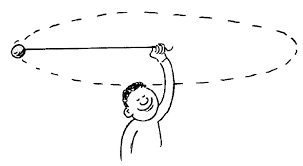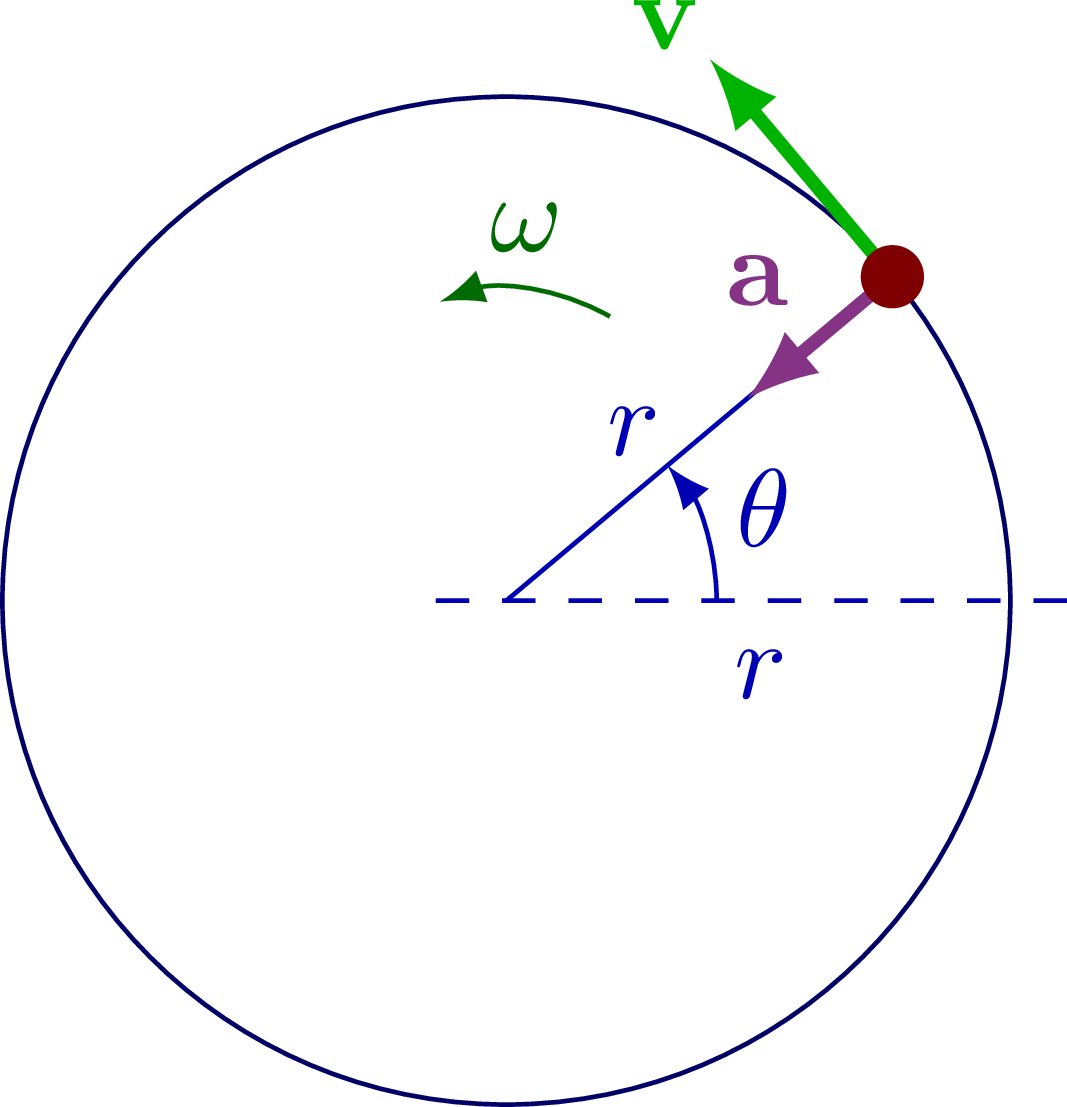# Circular Motion Study Guide

Introduction

We’ve all fantasized about riding a horse while wearing a nice cowboy hat and performing the lasso trick. The lasso trick is a superb illustration of a physical motion in and of itself. A lasso trick is, in fact, a famous illustration of circulatory motion. The continual circular motion of the hand keeps the rope moving and keeps it from dropping to the floor. Let’s take a closer look at circular motion.## What is Circular Motion?

• Circular motion’s definition says that this is the motion of an object along a circular route while spinning.
• Also, unlike linear motion, the direction of motion changes continuously in the case of the circular motion of a body.
• As a result, angular variables can be used to characterize circular motion.
• The angular variable in a circular motion is as:## Angular Variables

### Angular Displacement

• It’s the angle at which a rotating object turns in one unit of time.
• Theta (𝜃) is the symbol for it, and it is expressed in radians.

### Angular Velocity

• It’s the rate at which a particle’s angular displacement changes in a circular motion.
• The unit of angular velocity is rad/s.
• A particle in circular motion has linear velocity and matching linear speed in addition to angular velocity and angular speed.

### Angular Acceleration

• It is defined as the rate at which the rotating particle’s angular velocity changes.
• It is expressed in rad/s2.

The common circular motion formulas are

ω = lim∆t→0 (∆θ/∆t) = dθ/dt ,

α = dω/dt = d2θ/dt2,

ar = V2/R

Where ω is the angular velocity,

t is the time, θ is angular displacement,

α is the angular acceleration,

ar is the angular acceleration,

V is the velocity and

R is the radius of the motion.

## Types of Circular Motion

Circular motion can be of two types: uniform or non-uniform circular motion.

• The angular rate of rotation and speed are constant in uniform circular motion, whereas the rate of rotation changes in non-uniform motion.
• The velocity vector changes direction at every point on the curve during circular motion. As a result, the radial component of acceleration is never zero.
• In the event of non-uniform circular motion, the tangential component might be positive or negative, and in the case of a uniform circular motion, it can be zero.

Movement of a giant wheel, satellites orbiting around earth and the motion of a merry-go-round are some of the circular motion examples.

# Summary

• The motion of an object along a circular route while spinning is called circular Motion.
• Angular variables like angular displacement, angular velocity and angular acceleration can be used to characterize circular motion.
• Circular motion can be of two types: uniform or non-uniform circular motion.

## FAQs

Q. What is circular motion?

Circular motion is the motion of an object along a circular route while spinning.

Q. Is uniform circular motion accelerated motion?

Yes, uniform circular motion is an accelerated motion.

Q. Give two examples of circular motion.

Twirling a lasso and satellites orbiting around earth are two examples of circular motion.

We hope you enjoyed studying this lesson and learned something cool about Circular Motion! Join our Discord community to get any questions you may have answered and to engage with other students just like you! Don’t forget to download our App to experience our fun, VR classrooms – we promise, it makes studying much more fun!😎

]]>